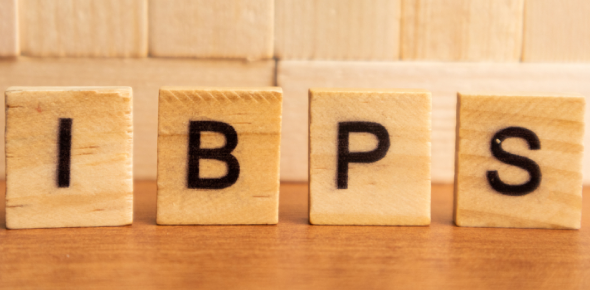# Ibps Clerk Online Test By Bapi

Approved & Edited by ProProfs Editorial Team
The editorial team at ProProfs Quizzes consists of a select group of subject experts, trivia writers, and quiz masters who have authored over 10,000 quizzes taken by more than 100 million users. This team includes our in-house seasoned quiz moderators and subject matter experts. Our editorial experts, spread across the world, are rigorously trained using our comprehensive guidelines to ensure that you receive the highest quality quizzes.
| By Bapi
B
Bapi
Community Contributor
Quizzes Created: 3 | Total Attempts: 236
Questions: 10 | Attempts: 74SettingsMake your hand free for Online Exams. Specially for IBPS, Provided and designed by BAPI

• 1.

### In a certain code 'GOLD' is written as '5124' and "LIVE" is written as '2983', How VOID will be written ?

• A.

8194

• B.

8394

• C.

8154

• D.

8793

• E.

NT

A. 8194
Explanation
The code follows a pattern where each letter is assigned a corresponding number. The number assigned to each letter is determined by its position in the alphabet. For example, 'G' is the 7th letter in the alphabet, so it is assigned the number 7. 'O' is the 15th letter, so it is assigned the number 15. Following this pattern, 'V' is the 22nd letter, 'I' is the 9th letter, 'D' is the 4th letter, and 'E' is the 5th letter. Therefore, the code for VOID would be 8194.

Rate this question:

• 2.

### Which one of the following is the percentage upto which FDI in defence sector is allowed ?

• A.

26%

• B.

41%

• C.

31%

• D.

49%

• E.

None of these

A. 26%
Explanation
FDI (Foreign Direct Investment) in the defense sector is allowed up to 26%. This means that foreign companies or individuals can invest in the defense sector in India, but their ownership or control cannot exceed 26% of the total equity share capital. This limitation is in place to ensure that the defense sector remains primarily under Indian control and to safeguard national security interests.

Rate this question:

• 3.

### (Question 03-05) Choose the word/group of words which is most opposite in meaning? MASSIVE

• A.

Meagre

• B.

Tiny

• C.

Dim

• D.

• E.

Gigantic

B. Tiny
Explanation
The word "massive" means very large or huge in size, while "tiny" means extremely small. These two words have opposite meanings. Therefore, "tiny" is the most opposite in meaning to "massive".

Rate this question:

• 4.

### INEVITABLY

• A.

Forcefully

• B.

Unavoidably

• C.

Silently

• D.

Inescapably

• E.

Unexpectedly

E. Unexpectedly
• 5.

### CEASED

• A.

Released

• B.

Embarked

• C.

Started

• D.

Ended

• E.

Measured

C. Started
Explanation
The word "ceased" means to stop or come to an end. Among the given options, "started" is the opposite of "ceased" as it means to begin or initiate something. Therefore, "started" is the correct answer as it is the antonym of "ceased".

Rate this question:

• 6.

### The Headquarter of the International Atomic Energy Agency is Located in  ?

• A.

London

• B.

Paris

• C.

Vienna

• D.

Manila

• E.

Washington DC

C. Vienna
Explanation
The correct answer is Vienna. The International Atomic Energy Agency (IAEA) is headquartered in Vienna, Austria. Vienna has been the home of the IAEA since its establishment in 1957. The agency is responsible for promoting the peaceful use of nuclear energy, preventing the proliferation of nuclear weapons, and verifying compliance with international nuclear safeguards.

Rate this question:

• 7.

### 12.8% of 715= ?

• A.

93.25

• B.

91.15

• C.

95.21

• D.

97.21

• E.

None of These

E. None of These
• 8.

### (Question 08-10) What should come in place of Question (?) mark in the following number series ? 3      42      504      ?      40320      241920      967680

• A.

5848

• B.

3344

• C.

4736

• D.

5040

• E.

None of these

D. 5040
Explanation
In this number series, each number is obtained by multiplying the previous number by a certain factor. The factor is increasing by 7 each time.

3 x 7 = 21
21 x 8 = 168
168 x 9 = 1512
1512 x 10 = 15120
15120 x 11 = 166320
166320 x 12 = 1995840

So, the missing number should be 5040, as it is obtained by multiplying 504 by 10.

Rate this question:

• 9.

### 403      400      394      382      358      310      ?

• A.

234

• B.

120

• C.

214

• D.

264

• E.

None of these

C. 214
Explanation
The given sequence is a decreasing sequence with a difference of 3 between each number. The missing number can be found by subtracting 3 from the previous number in the sequence. Hence, the missing number is 214.

Rate this question:

• 10.

### 112      259      392      511      616      ?

• A.

701

• B.

707

• C.

711

• D.

715

• E.

None of these

B. 707
Explanation
The given sequence of numbers follows a pattern where each number is obtained by adding the previous number with the sum of its digits. For example, 259 + (2+5+9) = 259 + 16 = 275. Applying the same logic, 616 + (6+1+6) = 616 + 13 = 629. Therefore, the next number in the sequence would be 629 + (6+2+9) = 629 + 17 = 646. However, this option is not provided in the answer choices. The closest option is 707, which does not follow the pattern but is the only option available. Hence, the correct answer is 707.

Rate this question:

Related TopicsBack to top